Hostname: page-component-546b4f848f-hhr79 Total loading time: 0 Render date: 2023-06-03T06:05:44.866Z Has data issue: false Feature Flags: { "useRatesEcommerce": true } hasContentIssue false

# The role of SPICA-like missions and the Origins Space Telescope in the quest for heavily obscured AGN and synergies with Athena

Published online by Cambridge University Press:  23 July 2021

*
Author for correspondence: L. Barchiesi, E-mail: luigi.barchiesi2@unibo.it

## Abstract

In the black hole (BH)–galaxy co-evolution framework, most of the star formation (SF) and the BH accretion are expected to take place in highly obscured conditions. The large amount of gas and dust absorbs most of the UV-to-soft-X radiation and re-emits it at longer wavelengths, mostly in the IR. Thus, obscured active galactic nuclei (AGN) are very difficult to identify in optical or X-ray bands but shine bright in the IR. Moreover, X-ray background (XRB) synthesis models predict that a large fraction of the yet-unresolved XRB is due to the most obscured (Compton thick, CT: N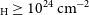$_{\text{H}}\ge 10^{24} \,\mathrm{cm}^{-2}$ ) of these AGN. In this work, we investigate the synergies between putative IR missions [using SPace Infrared telescope for Cosmology and Astrophysics (SPICA), proposed for European Space Agency (ESA)/M5 but withdrawn in 2020 October, and Origins Space Telescope, OST, as ‘templates’] and the X-ray mission Athena (Advanced Telescope for High ENergy Astrophysics), which should fly in early 2030s, in detecting and characterising AGN, with a particular focus on the most obscured ones. Using an XRB synthesis model, we estimated the number of AGN and the number of those which will be detected in the X-rays by Athena. For each AGN, we associated an optical-to-Far InfraRed (FIR) spectral energy distribution (SED) from observed AGN with both X-ray data and SED decomposition and used these SEDs to check if the AGN will be detected by SPICA-like or OST at IR wavelengths. We expect that, with the deepest Athena and SPICA-like (or OST) surveys, we will be able to photometrically detect in the IR more than 90% of all the AGN (down to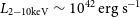$L_{2-10\text{keV}} \sim 10^{42}\,\mathrm{erg\ s}^{-1}$ and up to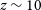$z \sim 10$ ) predicted by XRB synthesis modeling, and we will detect at least half of them in the X-rays. The spectroscopic capabilities of the OST can provide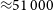${\approx}51\,000$ and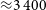${\approx}3\,400$ AGN spectra with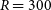$R= 300$ at 25–588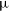$\unicode[Times]{x03BC}$ m in the wide and deep surveys, respectively, the last one up to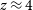$z\approx 4$ . Athena will be extremely powerful in detecting and discerning moderate- and high-luminosity AGN, allowing us to properly select AGN even when the mid-IR torus emission is ‘hidden’ by the host galaxy contribution. We will constrain the intrinsic luminosity and the amount of obscuration for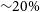$\sim\!20\%$ of all the AGN (and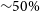$\sim\!50\%$ of those with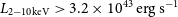$L_{2-10\text{keV}} > 3.2 \times 10^{43}\,\mathrm{erg\ s}^{-1}$ ) using the X-ray spectra provided by Athena WFI. We find that the most obscured and elusive CT-AGN will be exquisitely sampled by SPICA-like mission or OST and that Athena will allow a fine characterisation of the most luminous ones. This will provide a significant step forward in the process of placing stronger constraints on the yet-unresolved XRB and investigating the BH accretion rate evolution up to very high redshift (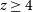$z \ge 4$ ).

Type
Research Article
© The Author(s), 2021. Published by Cambridge University Press on behalf of the Astronomical Society of Australia

## Access options

Get access to the full version of this content by using one of the access options below. (Log in options will check for institutional or personal access. Content may require purchase if you do not have access.)

## References

Aird, J., et al. 2015, ApJ, 815, 66 CrossRefGoogle Scholar
Aird, J., et al. 2010, MNRAS, 401, 2531 CrossRefGoogle Scholar
Ananna, T. T., et al. 2019, ApJ, 871, 240 CrossRefGoogle Scholar
André, P., et al. 2019, Probing the cold magnetized Universe with SPICA-POL (B-BOP)Google Scholar
Armus, L., et al. 2006, ApJ, 640, 204 CrossRefGoogle Scholar
Armus, L., et al. 2007, ApJ, 656, 148 CrossRefGoogle Scholar
Arnaud, K. A. 1996, in Astronomical Society of the Pacific Conference Series, Vol. 101, Astronomical Data Analysis Software and Systems V, ed. Jacoby, G. H., & Barnes, J., 17 Google Scholar
Assef, R. J., et al. 2013, ApJ, 772, 26 CrossRefGoogle Scholar
Ballantyne, D. R., Draper, A. R., Madsen, K. K., Rigby, J. R., & Treister, E. 2011, ApJ, 736, 56 CrossRefGoogle Scholar
Barai, P., et al. 2018, MNRAS, 473, 4003 CrossRefGoogle Scholar
Barcons, X., et al. 2017, Astronomische Nachrichten, 338, 153 CrossRefGoogle Scholar
Barret, D., et al. 2018, in Society of Photo-Optical Instrumentation Engineers (SPIE) Conference Series, Vol. 10699, Space Telescopes and Instrumentation 2018: Ultraviolet to Gamma Ray, 106991G Google Scholar
Battersby, C., et al. 2018, NatAs, 2, 596 Google Scholar
Bavdaz, M., et al. 2018, in Society of Photo-Optical Instrumentation Engineers (SPIE) Conference Series, Vol. 10699, Space Telescopes and Instrumentation 2018: Ultraviolet to Gamma Ray, 106990X Google Scholar
Berta, S., et al. 2013, A&A, 551, A100 Google Scholar
Bisigello, L., et al. 2020, arXiv e-prints, arXiv:2011.07074 Google Scholar
Bonato, M., et al. 2019, PASA, 36, e017 CrossRefGoogle Scholar
Brightman, M., & Nandra, K. 2011, MNRAS, 413, 1206 CrossRefGoogle Scholar
Brightman, M., & Ueda, Y. 2012, MNRAS, 423, 702 CrossRefGoogle Scholar
Burlon, D., et al. 2011, ApJ, 728, 58 CrossRefGoogle Scholar
Cash, W. 1979, ApJ, 228, 939 CrossRefGoogle Scholar
Castelló-Mor, N., et al. 2013, A&A, 556, A114 Google Scholar
Civano, F., et al. 2016, ApJ, 819, 62 CrossRefGoogle Scholar
Comastri, A., Gilli, R., Marconi, A., Risaliti, G., & Salvati, M. 2015, A&A, 574, L10 Google Scholar
Comastri, A., et al. 2011, A&A, 526, L9 Google Scholar
Comastri, A., Setti, G., Zamorani, G., & Hasinger, G. 1995, A&A, 296, 1 Google Scholar
Del Moro, A., et al. 2017, FASS, 4, 67 Google Scholar
Del Moro, A., et al. 2014, ApJ, 786, 16 CrossRefGoogle Scholar
Delvecchio, I., et al. 2014, MNRAS, 439, 2736 CrossRefGoogle Scholar
Delvecchio, I., et al. 2015, MNRAS, 449, 373 CrossRefGoogle Scholar
Di Matteo, T., Springel, V., & Hernquist, L. 2005, Natur, 433, 604 CrossRefGoogle Scholar
Dowell, C. D., et al. 1998, ApJ, 504, 588 CrossRefGoogle Scholar
Duras, F., et al. 2020, A&A, 636, A73 Google Scholar
Elvis, M., et al. 2009, ApJs, 184, 158 CrossRefGoogle Scholar
Feltre, A., Charlot, S., & Gutkin, J. 2016, MNRAS, 456, 3354 CrossRefGoogle Scholar
Fernández-Ontiveros, J. A., et al. 2016, ApJs, 226, 19 CrossRefGoogle Scholar
Ferrarese, L., & Merritt, D. 2000, ApJl, 539, L9 CrossRefGoogle Scholar
Feruglio, C., et al. 2015, A&A, 583, A99 Google Scholar
Fiore, F., et al. 2017, A&A, 601, A143 Google Scholar
Galitzki, N., et al. 2014, JAI, 03, 1440001 Google Scholar
García-Bernete, I., et al. 2016, MNRAS, 463, 3531 CrossRefGoogle Scholar
Gebhardt, K., et al. 2000, ApJl, 539, L13 CrossRefGoogle Scholar
Genzel, R., et al. 1998, ApJ, 498, 579 CrossRefGoogle Scholar
Georgantopoulos, I., et al. 2013, A&A, 555, A43 Google Scholar
Giacconi, R., et al. 2002, ApJs, 139, 369 CrossRefGoogle Scholar
Gilli, R. 2013, MSAI, 84, 647 Google Scholar
Gilli, R., Comastri, A., & Hasinger, G. 2007, A&A, 463, 79 Google Scholar
González, V., et al. 2011, ApJl, 735, L34 CrossRefGoogle Scholar
Goulding, A. D., et al. 2012, ApJ, 755, 5 CrossRefGoogle Scholar
Griffin, M. J., et al. 2010, A&A, 518, L3 Google ScholarPubMed
Gruppioni, C., et al. 2016, MNRAS, 458, 4297 CrossRefGoogle Scholar
Gruppioni, C., et al. 2020, A&A, 643, A8 Google Scholar
Gruppioni, C., et al. 2013, MNRAS, 432, 23 CrossRefGoogle Scholar
Harrison, F. A., et al. 2016, ApJ, 831, 185 CrossRefGoogle Scholar
Heckman, T. M., & Best, P. N. 2014, ARA&A, 52, 589 CrossRefGoogle Scholar
Hollenbach, D. J., & Tielens, A. G. G. M. 1999, RvMP, 71, 173 Google Scholar
Hopkins, P. F., et al. 2006, ApJs, 163, 1 CrossRefGoogle Scholar
Hopkins, P. F., Richards, G. T., & Hernquist, L. 2007, ApJ, 654, 731 CrossRefGoogle Scholar
Houck, J. R., et al. 2005, ApJl, 622, L105 CrossRefGoogle Scholar
Hughes, D. H., et al. 1998, Nature, 394, 241 CrossRefGoogle Scholar
Jaacks, J., Choi, J.-H., Nagamine, K., Thompson, R., & Varghese, S. 2012, MNRAS, 420, 1606 CrossRefGoogle Scholar
Kaneda, H., et al. 2017, PASA, 34, e059 CrossRefGoogle Scholar
Kormendy, J., & Richstone, D. 1995, ARA&A, 33, 581 CrossRefGoogle Scholar
La Caria, M. M., Vignali, C., Lanzuisi, G., Gruppioni, C., & Pozzi, F. 2019, MNRAS, 487, 1662 CrossRefGoogle Scholar
Laigle, C., et al. 2016, ApJs, 224, 24 CrossRefGoogle Scholar
Lamastra, A., et al. 2013, A&A, 559, A56 Google Scholar
Lansbury, G. B., et al. 2017a, ApJ, 846, 20 CrossRefGoogle Scholar
Lansbury, G. B., et al. 2017b, ApJ, 836, 99 CrossRefGoogle Scholar
Lanzuisi, G., et al. 2013, MNRAS, 431, 978 CrossRefGoogle Scholar
Lanzuisi, G., et al. 2017, A&A, 602, A123 Google Scholar
Lanzuisi, G., et al. 2015, A&A, 573, A137 Google Scholar
Lapi, A., et al. 2018, ApJ, 857, 22 CrossRefGoogle Scholar
Leger, A., D’Hendecourt, L., & Defourneau, D. 1989, A&A, 216, 148 Google Scholar
Lehmer, B. D., et al. 2012, ApJ, 752, 46 CrossRefGoogle Scholar
Li, H., Dowell, C. D., Kirby, L., Novak, G., & Vaillancourt, J. E. 2008, Appl. Opt., 47, 422 CrossRefGoogle Scholar
Luo, B., et al. 2008, ApJs, 179, 19 CrossRefGoogle Scholar
Lusso, E., et al. 2012, MNRAS, 425, 623 CrossRefGoogle Scholar
Lusso, E., et al. 2010, A&A, 512, A34 Google Scholar
Lutz, D., Spoon, H. W. W., Rigopoulou, D., Moorwood, A. F. M., & Genzel, R. 1998, ApJl, 505, L103 CrossRefGoogle Scholar
Lutz, D., et al. 2003, A&A, 409, 867 Google Scholar
Magnelli, B., et al. 2013, A&A, 553, A132 Google Scholar
Marchesi, S., et al. 2016, ApJ, 817, 34 CrossRefGoogle Scholar
Mateos, S., et al. 2012, MNRAS, 426, 3271 CrossRefGoogle Scholar
McCammon, D., et al. 2002, ApJ, 576, 188 CrossRefGoogle Scholar
Meidinger, N., Nandra, K., & Plattner, M. 2018, in Society of Photo-Optical Instrumentation Engineers (SPIE) Conference Series, Vol. 10699, Space Telescopes and Instrumentation 2018: Ultraviolet to Gamma Ray, 106991F Google Scholar
Meixner, M., et al. 2019, arXiv e-prints, arXiv:1912.06213 Google Scholar
Menci, N., Fiore, F., Puccetti, S., & Cavaliere, A. 2008, ApJ, 686, 219 CrossRefGoogle Scholar
Mordini, S., Spinoglio, L., & Fernández-Ontiveros, J. A. 2021, arXiv e-prints, arXiv:2105.04584 Google Scholar
Nakagawa, T., et al. 1998, Society of Photo-Optical Instrumentation Engineers (SPIE) Conference Series, Vol. 3356, HII/L2 Mission: Future Japanese Infrared Astronomical Mission, ed. Bely, P. Y., & Breckinridge, J. B., 462 CrossRefGoogle Scholar
Nakagawa, T., et al. 2014, Society of Photo-Optical Instrumentation Engineers (SPIE) Conference Series, Vol. 9143, The Next-Generation Infrared Astronomy Mission SPICA Under the New Framework, 91431I CrossRefGoogle Scholar
Nandra, K., et al. 2013, arXiv e-prints, arXiv:1306.2307 Google Scholar
O’Dowd, M. J., et al. 2009, ApJ, 705, 885 CrossRefGoogle Scholar
Page, M. J., Stevens, J. A., Ivison, R. J., & Carrera, F. J. 2004, ApJl, 611, L85 CrossRefGoogle Scholar
Peca, A., 2021, ApJ, 906, 90 CrossRefGoogle Scholar
Poglitsch, A., et al. 2010, A&A, 518, L2 Google Scholar
Polletta, M., et al. 2008, ApJ, 675, 960 CrossRefGoogle Scholar
Pozzi, F., et al. 2007, A&A, 468, 603 Google Scholar
Ricci, C., et al. 2015, ApJl, 815, L13 CrossRefGoogle Scholar
Riechers, D. A., et al. 2013, Nature, 496, 329 CrossRefGoogle Scholar
Rodriguez, L., et al. 2018, JLTP, 193, 449 CrossRefGoogle Scholar
Roelfsema, P. R., et al. 2018, PASA, 35, e030 CrossRefGoogle Scholar
Rowan-Robinson, M., et al. 1997, MNRAS, 289, 490 CrossRefGoogle Scholar
Rowan-Robinson, M., 2016, MNRAS, 461, 1100 CrossRefGoogle Scholar
Schreiber, C., et al. 2015, A&A, 575, A74 Google Scholar
Scoville, N., et al. 2007, ApJs, 172, 1 CrossRefGoogle Scholar
Shankar, F., Weinberg, D. H., & Miralda-Escudé, J. 2009, ApJ, 690, 20 CrossRefGoogle Scholar
Shankar, F., Weinberg, D. H., & Miralda-Escudé, J. 2013, MNRAS, 428, 421 CrossRefGoogle Scholar
Shi, Y., et al. 2006, ApJ, 653, 127 CrossRefGoogle Scholar
Sijacki, D., et al. 2015, MNRAS, 452, 575 CrossRefGoogle Scholar
Silk, J., & Rees, M. J. 1998, A&A, 331, L1 Google Scholar
Simmonds, C., Buchner, J., Salvato, M., Hsu, L. T., & Bauer, F. E. 2018, A&A, 618, A66 Google Scholar
Smith, J. D. T., et al. 2007, ApJ, 656, 770 CrossRefGoogle Scholar
Smith, R. N., Tombesi, F., Veilleux, S., Lohfink, A. M., & Luminari, A. 2019, ApJ, 887, 69 CrossRefGoogle Scholar
Spergel, D. N., et al. 2003, ApJs, 148, 175 CrossRefGoogle Scholar
Spinoglio, L., et al. 2017, PASA, 34, e057 CrossRefGoogle Scholar
Spinoglio, L., & Malkan, M. A. 1992, ApJ, 399, 504 CrossRefGoogle Scholar
Spinoglio, L., et al. 2021, PASA, 38, e021 CrossRefGoogle Scholar
Sturm, E., et al. 2006, ApJ, 642, 81 CrossRefGoogle Scholar
Swinyard, B., et al. 2009, ExA, 23, 193 Google Scholar
Teplitz, H. I., et al. 2006, ApJl, 638, L1 CrossRefGoogle Scholar
Thomas, N., Davé, R., Anglés-Alcázar, D., & Jarvis, M. 2019, MNRAS, 487, 5764 CrossRefGoogle Scholar
Tombesi, F., et al. 2015, Natur, 519, 436 CrossRefGoogle Scholar
Tommasin, S., Spinoglio, L., Malkan, M. A., & Fazio, G. 2010, ApJ, 709, 1257 CrossRefGoogle Scholar
Tommasin, S., et al. 2008, ApJ, 676, 836 CrossRefGoogle Scholar
Tozzi, P., et al. 2006, A&A, 451, 457 Google Scholar
Treister, E., Urry, C. M., & Virani, S. 2009, ApJ, 696, 110 CrossRefGoogle Scholar
Ueda, Y., Akiyama, M., Hasinger, G., Miyaji, T., & Watson, M. G. 2014, ApJ, 786, 104 CrossRefGoogle Scholar
Vignali, C., et al. 2011, MNRAS, 416, 2068 CrossRefGoogle Scholar
Vignali, C., et al. 2009, MNRAS, 395, 2189 CrossRefGoogle Scholar
Vito, F., et al. 2018, MNRAS, 473, 2378 CrossRefGoogle Scholar
Vito, F., et al. 2014, MNRAS, 445, 3557 CrossRefGoogle Scholar
Voit, G. M. 1992, MNRAS, 258, 841 CrossRefGoogle Scholar
Volonteri, M., Dubois, Y., Pichon, C., & Devriendt, J. 2016, MNRAS, 460, 2979 CrossRefGoogle Scholar
Weedman, D., et al. 2006, ApJ, 653, 101 CrossRefGoogle Scholar
Wu, Y., Charmandaris, V., Huang, J., Spinoglio, L., & Tommasin, S. 2009, ApJ, 701, 658 CrossRefGoogle Scholar
Xue, Y. Q. 2017, NewAR, 79, 59 CrossRefGoogle Scholar
Xue, Y. Q., et al. 2011, ApJs, 195, 10 CrossRefGoogle Scholar
Yan, L., et al. 2007, ApJ, 658, 778 CrossRefGoogle Scholar
Yang, G., et al. 2020, MNRAS, 491, 740 CrossRefGoogle Scholar
Zappacosta, L., et al. 2018, A&A, 618, A28 Google Scholar